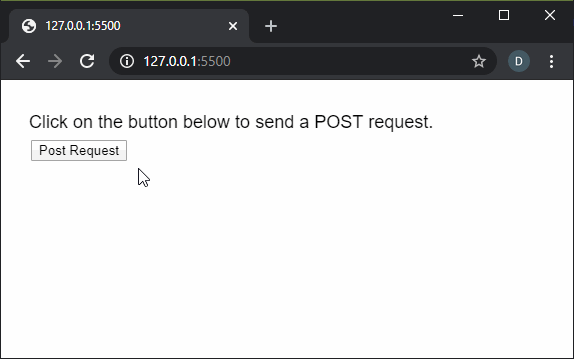# p5.js | httpPost() Function

• Last Updated : 02 May, 2022

The httpPost() function in p5.js is used to execute an HTTP POST request. The datatype returned is automatically guessed by p5 based on the URL, when it is not specified. The data returned could be loaded in the preload() function so that it can be accessed immediately in the program. Syntax:

`httpPost( path, [datatype], [data], [callback], [errorCallback] )`

OR

`httpPost( path, data, [callback], [errorCallback] )`

OR

`httpPost( path, callback, [errorCallback] )`

Parameters: This function accept five parameters as mentioned above and described below:

• path: It is a string that denotes the path of the URL or file to load.
• datatype: It is a string that specifies the type of data that will be received. It can have the values of “json”, “jsonp”, “xml”, or “text”. It will default to ‘text’ is no parameter is specified. It is an optional parameter.
• data: It is an Object or a Boolean value that specifies the parameter data passed with the request.
• callback: It is a function which is called when this function executes successfully. The first argument for this function is the data returned from the API. It is an optional parameter.
• errorCallback: It is a function which is called if there is any error in executing the function. The first argument for this function is the error response. It is an optional parameter.

Return Value: It returns a promise that can be resolved with the data when the operation completes successfully, or be rejected when an error takes place.

Below examples illustrates the httpPost() function in p5.js:

Example 1:

## javascript

 `function` `setup() {``  ``createCanvas(550, 200);``  ``textSize(18);`` ` `  ``text("Click on the button below"+``       ``" to send a POST request.", 20, 40);`` ` `  ``postBtn = createButton("Post Request");``  ``postBtn.position(30, 60);``  ``postBtn.mouseClicked(postRequest);``}`` ` `function` `postRequest() {`` ` `  ``// Do a POST request to the test API``  ``let api_url = ``'https://reqres.in/api/users'``;`` ` `  ``// Example POST data``  ``let postData = { id: 1, name: "Sam",``                  ``email: "sam@samcorp.com" };`` ` `  ``httpPost(api_url, ``'json'``, postData, ``function` `(response) {``    ``text("Data returned from API", 20, 100);`` ` `    ``text("The ID ``in` `the data is: "``         ``+ response.id, 20, 140);``    ``text("The Name ``in` `the data is: "``         ``+ response.name, 20, 160);``    ``text("The Email ``in` `the data is: "``         ``+ response.email, 20, 180);``  ``});``}`

Output:Example 2:

## javascript

 `function` `setup() {``  ``createCanvas(550, 200);``  ``textSize(18);`` ` `  ``// Do a POST request to the test API``  ``let api_url =``      ``'https://reqres.in/api/users'``;`` ` `  ``let postData = { id: 1, name: "James",``                  ``email: "james@james.j.com" };`` ` `  ``httpPost(api_url, ``'json'``, postData,``           ``onSuccessfulFetch, onErrorFetch);``}`` ` `function` `onSuccessfulFetch(response) {``  ``text("Data returned from API", 20, 60);`` ` `  ``text("The ID ``in` `the data is: "``       ``+ response.id, 20, 100);``  ``text("The Name ``in` `the data is: "``       ``+ response.name, 20, 120);``  ``text("The Email ``in` `the data is: "``       ``+ response.email, 20, 140);``}`` ` `function` `onErrorFetch() {``  ``text("There was an error doing"+``       ``" the request.", 20, 60);``}`

Output:Online editor: https://editor.p5js.org/

Reference: https://p5js.org/reference/#/p5/httpPost

My Personal Notes arrow_drop_up